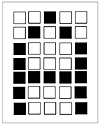# 83.C++ Neural Networks and Fuzzy Logic by Valluru B. Rao M&T Books, IDG Books Worldwide, Inc. ISBN: 1558515526   Pub Date: 06/01/95

## Chapter 12Application to Pattern Recognition

### Using the Kohonen Feature Map

In this chapter, you will use the Kohonen program developed in Chapter 11 to recognize patterns. You will modify the Kohonen program for the display of patterns.

#### An Example Problem: Character Recognition

The problem that is presented in this chapter is to recognize or categorize alphabetic characters. You will input various alphabetic characters to a Kohonen map and train the network to recognize these as separate categories. This program can be used to try other experiments that will be discussed at the end of this chapter.

Representing Characters

Each character is represented by a 5×7 grid of pixels. We use the graphical printing characters of the IBM extended ASCII character set to show a grayscale output for each pixel. To represent the letter A, for example, you could use the pattern shown in Figure 12.1. Here the blackened boxes represent value 1, while empty boxes represent a zero. You can represent all characters this way, with a binary map of 35 pixel values.Figure 12.1  Representation of the letter A with a 5×7 pattern.

The letter A is represented by the values:

` 0 0 1 0 0 0 1 0 1 0 1 0 0 0 1 1 0 0 0 1 1 1 1 1 1 1 0 0 0 1 1 0 0 0 1 `

For use in the Kohonen program, we need to serialize the rows, so that all entries appear on one line.

For the characters A and X you would end up with the following entries in the input file, input.dat:

` 0 0 1 0 0  0 1 0 1 0  1 0 0 0 1  1 0 0 0 1  1 1 1 1 1  1 0 0 0 1  1 0 0 0 1  << the letter A 1 0 0 0 1  0 1 0 1 0  0 0 1 0 0  0 0 1 0 0  0 0 1 0 0  0 1 0 1 0  1 0 0 0 1  << the letter X `

Monitoring the Weights

We will present the Kohonen map with many such characters and find the response in output. You will be able to watch the Kohonen map as it goes through its cycles and learns the input patterns. At the same time, you should be able to watch the weight vectors for the winner neurons to see the pattern that is developing in the weights. Remember that for a Kohonen map the weight vectors tend to become aligned with the input vectors. So after a while, you will notice that the weight vector for the input will resemble the input pattern that you are categorizing.

Representing the Weight Vector

Although on and off values are fine for the input vectors mentioned, you need to see grayscale values for the weight vector. This can be accomplished by quantizing the weight vector into four bins, each represented by a different ASCII graphic character, as shown in Table 12.1.

Table 12.1 Quantizing the Weight Vector

<= 0 White rectangle (space)
0 < weight <= 0.25 Light-dotted rectangle
0.25 < weight <= 0.50 Medium-dotted rectangle
0.50 < weight <= 0.75 Dark-dotted rectangle
weight > 0.75 Black rectangle

The ASCII values for the graphics characters to be used are listed in Table 12.2.

Table 12.2 ASCII Values for Rectangle Graphic Characters

White rectangle 255
Light-dotted rectangle 176
Medium-dotted rectangle 177
Dark-dotted rectangle 178
Black rectangle 219

### C++ Code Development

The changes to the Kohonen program are relatively minor. The following listing indicates these changes.

#### Changes to the Kohonen Program

The first change to make is to the Kohonen_network class definition. This is in the file, layerk.h, shown in Listing 12.1.

Listing 12.1 Updated layerk.h file

` class Kohonen_network { private:        layer *layer_ptr;        int layer_size;        int neighborhood_size; public:        Kohonen_network();        ~Kohonen_network();        void get_layer_info();        void set_up_network(int);        void randomize_weights();        void update_neigh_size(int);        void update_weights(const float);        void list_weights();        void list_outputs();        void get_next_vector(FILE *);        void process_next_pattern();        float get_win_dist();        int get_win_index();        void display_input_char();        void display_winner_weights(); }; `C++ Neural Networks and Fuzzy Logic
ISBN: 1558515526
EAN: 2147483647
Year: 1995
Pages: 139

Similar book on Amazon